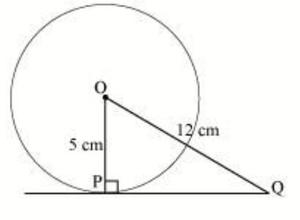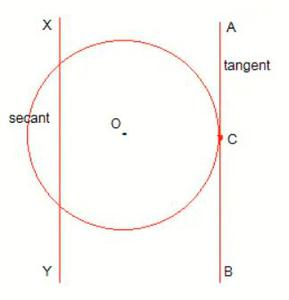# Class 10 NCERT Solutions – Chapter 10 Circles – Exercise 10.1

• Last Updated : 03 May, 2021

### Question 1. How many tangents can a circle have?

Solution:

A circle can have an infinite number of tangents because it has a infinite number of points on its circumference and each point can have its individual tangent.

### (iv) The common point of a tangent to a circle and the circle is called ____.

Solution:

(i) one

(ii) secant

(iii) two

(iv) Point of contact

### (A) 12 cm (B) 13 cm (C) 8.5 cm (D) √119 cm

Solution:

As we know that the line drawn from the centre of the circle to the tangent is perpendicular to the tangent at the point of the contact.Here OP is perpendicular to PQ.

Hence, the triangle OPQ is a Right angled Triangle, and we can apply Pythagoras Theorem in it.

PQ = √(OQ2OP2)

PQ = √(122 – 52)cm

PQ = √119 cm

Hence, Option D is the correct answer.

### Question 4. Draw a circle and two lines parallel to a given line such that one is a tangent and the other, a secant to the circle.

Solution:

Here AB is the tangent to the circle at point C and XY is the secant to the circle. Also, both lines are parallel.My Personal Notes arrow_drop_up